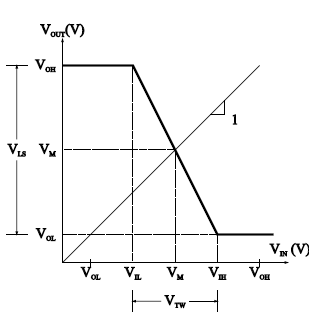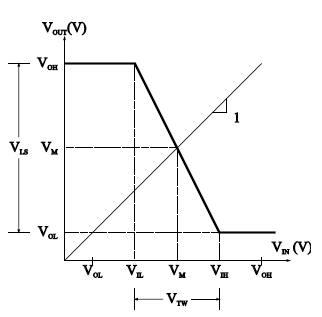### Inverter – Voltage Transfer Characteristic

Voltage Transfer Characteristic (VTC) for logic inverters have been standardized. VTC is the graph between Vout and VIN.
On vertical axis, VOH and VOL correspond to output high and output low voltage levels respectively. On the horizontal axis, VIL is input low voltage and VIH is the input high voltage.As the input voltage is increased from 0V, VIL is the maximum input voltage that provides a high output voltage (logical 1 output).
VIH is the minimum input voltage that provides a low output voltage (logical 0 output). VOH, VOL, VIL and VIH are referred to as the  critical voltages of the VTC.VOH > VIH
VOL < VIL
Midpoint Voltage:
Sometimes referred as Threshold voltage (Vth). The voltage at which Vout = VIN on VTC is referred as Midpoint voltage. Midpoint voltage can be found graphically by superimposing (the unity slope) Vout = VIN and finding its intersection  with the VTC.

Logic Swing and Transition Width
Logic Swing

The magnitude of voltage difference between the output high and low voltage levels.
VLS = VOH – VOL

Transition Width
The amount of voltage change that is required of the input voltage to cause a change in the output voltage from the high to the low level  (and vice versa).
VTW = VIH - VIL

Noise in Digital Circuits
Noise
Variations in the steady-state voltage levels of digital circuits (i.e. Logical 1 and logical 0 states) are undesirable and cause logic errors. This variation is termed as Noise.

Noise Margins
Voltage Noise Margin represents the safety margin
for the high and low voltage levels.
Noise voltages must have magnitudes less than the voltage noise margins.
VNMH = VOH – VIH
VNML = VIL – VOL

Noise Sensitivities
The effects of input variations are quantified in terms
of the noise sensitivities. The high noise sensitivity is defined as the difference
between input and midpoint voltage for VIN  at VOH.
The low noise sensitivity is defined as the difference between input and midpoint voltage for VIN at VOL.
VNSH = VOH – VM
VNSL = VM - VOL